﻿ Optimal Design of Step Stress Partially Accelerated Life Test under Progressive Type-II Censored Data with Random Removal for Gompertz DistributionPublications are Open
Access in this journal
Article Versions
Export Article
• Normal Style
• MLA Style
• APA Style
• Chicago Style
Research Article
Open Access Peer-reviewed

### Optimal Design of Step Stress Partially Accelerated Life Test under Progressive Type-II Censored Data with Random Removal for Gompertz Distribution

American Journal of Applied Mathematics and Statistics. 2019, 7(1), 37-42. DOI: 10.12691/ajams-7-1-6
Received November 12, 2018; Revised December 19, 2018; Accepted January 01, 2019

### Abstract

This paper deals with random removal of progressively type II censored data. The removal of the data is assumed to follow a binomial or a uniform distribution, and the life time testing is assumed to follow a Gompertz distribution. Parameters of these distributions are estimated using the Maximum Likelihood estimation procedure. Fisher information matrix is used to estimate the asymptotic mean squared error and to construct confidence intervals of model parameters. The optimal partially accelerated lifetime testing (PALT) is estimated by minimizing the Generalized Asymptotic Variance (GAV). Simulation study is performed to Clarification the statistical properties of the parameters. A simulation results reveal that for the fixed values of the parameters, the error and optimal time decrease with increasing sample size n; estimates of binomial are more stable with a relatively small error with increasing sample size; and the test design is robust and works well for binomial removal.

### 1. Introduction

Traditional life testing may show no failure or few failures of some highly reliable units because of some severe conditions (stresses) that may occur in the form of pressure, voltage, temperature, vibration, load, cycling rate, etc. In such situations life testing has to be performed at higher than usual conditions in order to obtain failures as fast as possible. The data collected under stresses or severe conditions should not be used to estimate the life distribution at normal use assumptions. There are three types of stress, a) step stress; b) progressive stress, and c) constant stress; Ismail, 1 when testing is conducted under stress, it is called either “Accelerated Life Testing (ALT)” or “Partially Accelerated Life Testing (PALT)”. In ‘Alt” testing, units are put under stress to reach more failure in short time, and the mathematical stress model is known; while in “PALT”, data can be extrapolated to normal use conditions, and the statistical model parameters could be estimated and step-stress could be applied. Nelson 2 proposed the step stress PALT (SS-PALT) where units are tested at normal use conditions within a specified time interval. Censored schemes arise when not all lifetime testing units are observed. When a unit does not fail within that time interval, it is put under stress, until failure or until the termination of the time interval (censored scheme). Rao 3 and Balakrishnan and Aggarwala 4 have mentioned that step stress saves money, effort and time. There are two censoring scheme types. Type I arises when the experiment continues up to a pre-specified time T and Type-II censoring scheme requires the experiment to continue until a pre-specified number of failures m ≤ n occur.

These two types do not allow for the removal of any unit until life testing is terminated. Nevertheless, this allowance may be desirable to compromise between at least one observation is sought and a reduced time interval for the experimentation. At this situation, progressive censoring is needed.

In the progressive Type II censoring scheme, the experimenter selects n (iid) units for life testing; and observes the occurrence of the first failure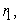at time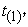and this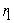is removed from testing. When the second failure occurs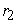at time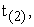one of the surviving units are randomly selected and removed from the test, the experiment terminates when the mth failure occurs at time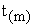and the remaining surviving units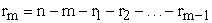are all removed from the Test Progressive type-II censoring scheme with fixed number of removals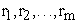is considered by Cohen 5 and Cohen and Norgaard 6. In some reliability experiments and when the number of removals is not fixed, a progressive censoring with random removals model could be considered 7, 8, 9. The random removal is assumed to follow the binomial distribution, and used to estimate the parameters of the reliability model.

Ismail 1 discusses step-stress partially-accelerated model. The model differs than the progressive type II censoring scheme in that the lifetime of the test follows the exponential distribution rather than the binomial.

This paper considers the step-stress partially-accelerated model, where the removals are assumed to follow the binomial and the life time-testing follows the Gompertz distribution. The optimal stress change time is also being determined by minimizing the generalized asymptotic variance of the MLE parameters. In Section 2, assumptions of the Gompretz distribution are given; Section 3 presents the assumptions of the partially accelerated model. Estimation of model parameters is given in Section 3; simulation study results are given in Section 4.

### 2. The Gompretz Distribution

The probability density function of the Gompretz distribution takes the form: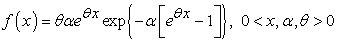(1)

And the cumulative distribution function is: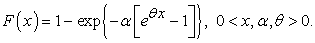(2)

The following assumptions are used:

1. n identical and independent units are selected for life testing.

2. The lifetime of each unit has Gompertz distribution.

The following steps are to be followed:

a) Each of the n units is first run under normal use condition. If it does not fail or remove from the test by a pre-specified time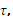. it is put under accelerated condition (stress).

b) At the ith failure a random number of the surviving units,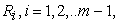are randomly selected and removed from the test.

c) Finally, at the mth failure the remaining surviving units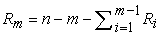are all removed from the test and the test is terminated.

The lifetime, say X, of a unit under SS-PALT can be rewritten as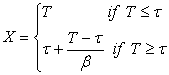Where, the pdf of X is given by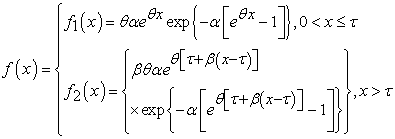(3)

In addition, the survival functions under normal and accelerate use conditions respectively is given by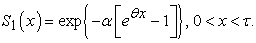(4)

And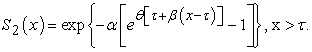(5)

### 3. Estimation of Parameters

Let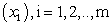, denote the observation obtained form a progressively type-II censored sample with random removals in a step-stress PALT. Here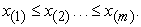Given a pre-determined number of removals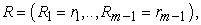the conditional likelihood function of the observations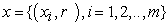takes the following form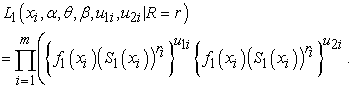(6)

Equations (1) and (3) are inserted in (6) and simplify, we get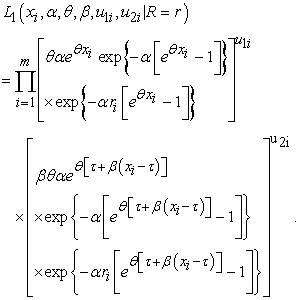(7)
3.1. Parameter Estimation with Binomial Removals

Given that the number of units removed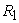from the test at each failure time follows a binomial distribution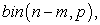and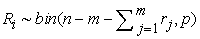for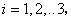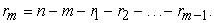Thus, the number of units removed at each failure time follows a binomial distribution such that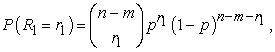And for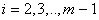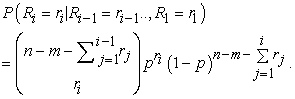(8)

The likelihood of the sample of size n is given as follows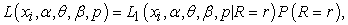(9)

Where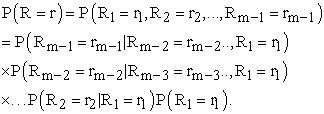That is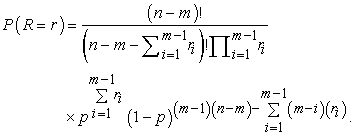(10)

The log-likelihood function [Eq. 10] can be written as follows: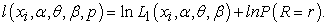(11)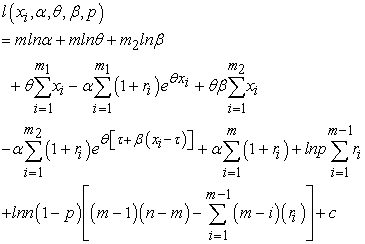(12)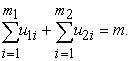To obtain the estimate of the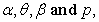the first partial derivatives with respect to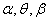and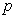of Equation (12) are obtained and equated to zero as follows: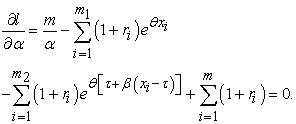(13)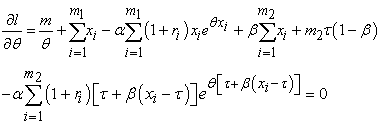(14)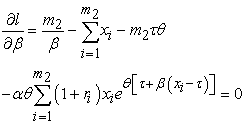(15)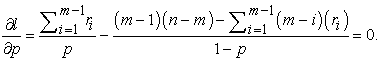(16)

From Equation (16),is estimated as follows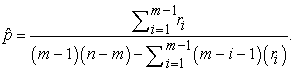(17)

There is no closed-from solution to this system of equations 13, 14 and 15. using the Newton-Raphson iteration method, for root finding.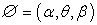is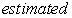Iteratively as follows: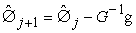(18)

where g is the vector of normal equations,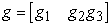and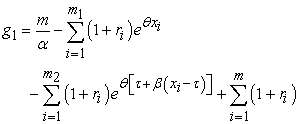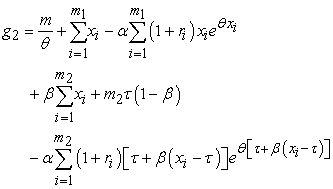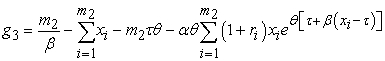and G [Equation 17] is the matrix of second derivatives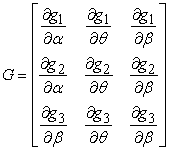(19)

Where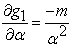(20)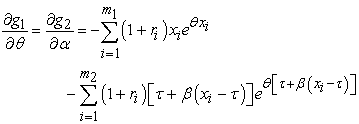(21)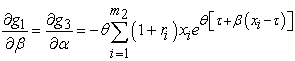(22)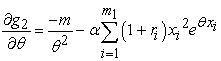(23)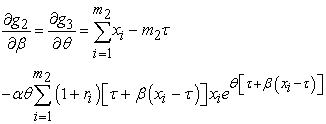(24)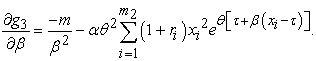(25)

Convergence of the Newton-Raphson algorithm for the estimates of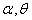and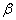depends on the tolerance limit change with each successive iteration, to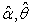and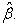Numerically inverting the above G matrix above, we easily obtain Fisher Information matrix, i.e.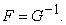The approximate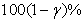two sided confidence intervals forandcan be, constructed as follows: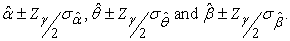3.2. Parameter Estimation with the Uniform Removals

The model assumes that removed units are independent and that the probability of each removed unit is the same, such that: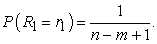(26)

And for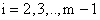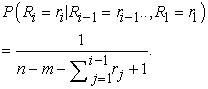The joint probability distribution of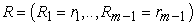is given by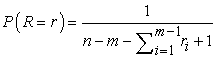(27)

where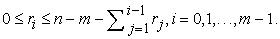The maximum likelihood estimators can be derived directly by maximizing the equations (12) and then solving for equations (13), (14) and (15).

3.3. D-optimality

Fisher's information matrix is used to determine t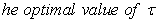in the SS-PALT type II progressive censoring scheme proposed criterion is based on the determinant of Fisher's information matrix. The generalized asymptotic variance (GAV) is the reciprocal of the determinant of Fisher's information matrix F 10, thus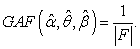(28)

The D-optimality criterion is the optimal value of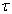that maximizes the determinant of the Fisher's information matrix F and minimizes the GAV.

### 4. Simulation Study

A simulation study is performed to study the properties of the estimators using ML method; the study involves the computation of Mean squared errors (MSEs), the construction of confidence intervals for different sample sizes; and the determination of, the optimal stress change time. The following steps were followed:

a)Value of n and m to be specified.

b)Value of the parameters were set as: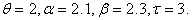.

c) A random sample with size n and censoring size m were generated, with random removals,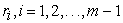from the random variable X given by (3).

d) Generate a group value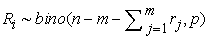and also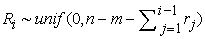where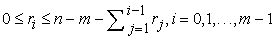ande) ML estimates were computed, for n= 20, 50, 80 and 100,

f) The mean squared error (MSE), the 95% confidence interval of parameters and the bias associated with the MLE of the parameters, optimal value of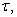the Optimal GAV of the MLEs of the model parameters are obtained numerically for each sample size.

### 5. Conclusions

The (SS-PALT) under progressive type-II censored data with binomial and uniform random removal assuming Gompertz distribution was studied. The Newton-Raphson method is applied to obtain the optimal stress-change time which minimizes the Generalized Asymptotic Variance (GAV).

The maximum Likelihood estimation procedure was used the estimation of model parameters, mean squared error and the optimum plan for the binomial and uniform removals for different sample size were computed and shown. Simulation results show that the error and the optimal time decrease as sample size increase, when parameters are fixed. that both the average value ofand the average value of GAV for type-II progressive censoring are getting close to those of complete sample with the bigger m and close faster for bigger n. Hence from the numerical result we can conclude that estimates of binomial are more stable with relatively small error with increasing sample size. Therefore, the test design obtained here is robust design and work well for binomial removal.

### References

  A. Ismail, (2009). Optimal Design of Step-Stress Life Test with Progressively type-II Censored Exponential Data, International Mathematical Forum, 4 , no 40, 1963-1976. In article  W. Nelson. (1990). Accelerated Life Testing: Statistical Models, Data Analysis and Test Plans, John Wiley and sons, New York. In article View Article  R. Rao, (1992), Equivalence of the tampered random variables and tampered failure rate models in ALT for a class of life distribution having the setting the clock back to zero property, Communication in Statistics – Theory and Methods, Vol. 21, No. 3, 647-664. In article View Article  N. Balakrishnan, and R. Aggarwala. (2000). Progressive Censoring: Theory, Methods, and Applications, Birkhauser, Boston. In article View Article  C. Cohen. (1963). Progressively Censored Samples in the Life Testing, Technometerics, 5, 327-339. In article View Article  C. Cohen and N. J. Norgaard. (1977). Progressively Censored Sampling in the Three Parameter Gamma Distribution, Technometerics, 19 , 333-340. In article View Article  H. K. Yuen. and S. K. (1996). Tse, Parameters Estimation for Weibull distributed Lifetime under progressive Censoring with random removals, Journal of Statistical Computation and Simulation, 55, 57-71. In article View Article  C. Wu, S. F. Wu, and, H. Y. Chan. (2004). MLE and the Estimated Expected Test Time for Pareto Distribution under Progressive Censoring Data, International Journal of Information Management Sciences, 15(3), 29-42. In article  Tse, S. K., Yang, C. and. Yuen, H. K., 2000. Statistical Analysis of Weibull Distributed Lifetime Data under Type-II Progressive Censoring with Binomial Removals. Journal of Applied Statistics, vol. 27, no. 8, pp. 1033-1043. In article View Article  S. Bai, J. G. Kim and Y. R. Chun, (1993). Design of Failure-Censored Accelerated Life-Test Sampling Plans for Lognormal and Weibull Distributions, Eng. Opt. Vol. 21, 197-212. In article View ArticleThis work is licensed under a Creative Commons Attribution 4.0 International License. To view a copy of this license, visit http://creativecommons.org/licenses/by/4.0/